# Maharashtra Board 11th Commerce Maths Solutions Chapter 6 Permutations and Combinations Ex 6.6

Balbharati Maharashtra State Board 11th Commerce Maths Solution Book Pdf Chapter 6 Permutations and Combinations Ex 6.6 Questions and Answers.

## Maharashtra State Board 11th Commerce Maths Solutions Chapter 6 Permutations and Combinations Ex 6.6

Question 1.
Find the value of
(i) 15C4
Solution: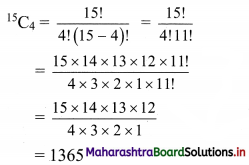(ii) 80C2
Solution: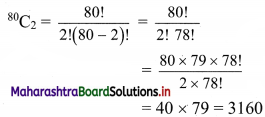(iii) 15C4 + 15C5
Solution: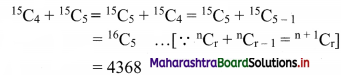(iv) 20C1619C16
Solution: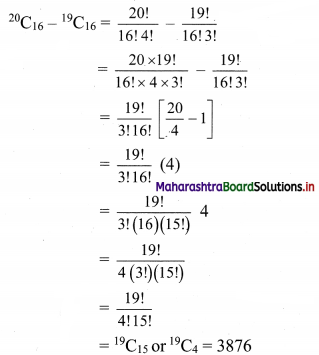Question 2.
Find n if
(i) 6P2 = n 6C2
Solution: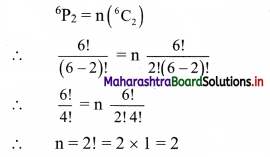(ii) 2nC3 : nC2 = 52 : 3
Solution: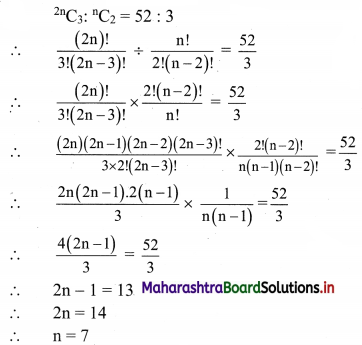(iii) nCn-3 = 84
Solution:
nCn-3 = 84
∴ $$\frac{n !}{(n-3) ![n-(n-3)] !}$$ = 84
∴ $$\frac{\mathrm{n}(\mathrm{n}-1)(\mathrm{n}-2)(\mathrm{n}-3) !}{(\mathrm{n}-3) ! \times 3 !}$$ = 84
∴ n(n – 1) (n – 2) = 84 × 6
∴ n(n – 1) (n – 2) = 9 × 8 × 7
Comparing on both sides, we get
∴ n = 9Question 3.
Find r if 14C2r : 10C2r-4 = 143 : 10
Solution: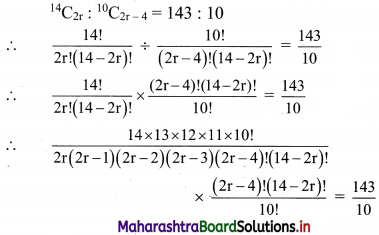∴ $$\frac{14 \times 13 \times 12 \times 11}{2 \mathrm{r}(2 \mathrm{r}-1) \times(2 \mathrm{r}-2)(2 \mathrm{r}-3)}=\frac{143}{10}$$
∴ 2r(2r – 1)(2r – 2)(2r – 3) = 14 × 12 × 10
∴ 2r(2r – 1)(2r – 2)(2r – 3) = 8 × 7 × 6 × 5
Comparing on both sides, we get
∴ r = 4

Question 4.
Find n and r if.
(i) nPr = 720 and nCn-r = 120
Solution: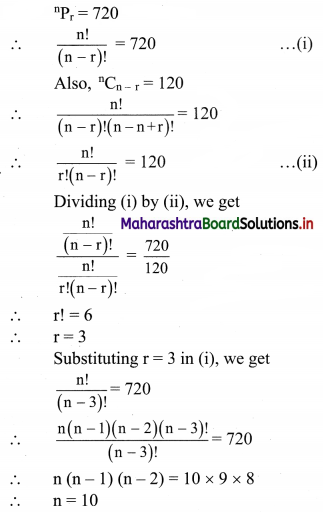(ii) nCr-1 : nCr : nCr+1 = 20 : 35 : 42
Solution: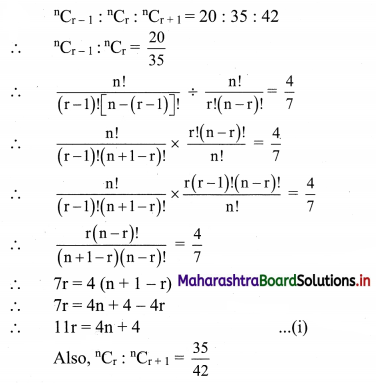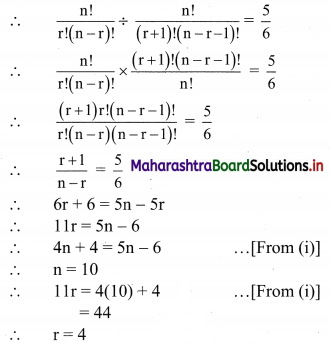Question 5.
If nPr = 1814400 and nCr = 45, find r.
Solution: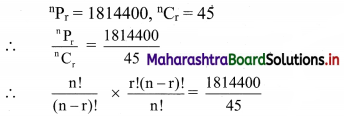∴ r! = 40320
∴ r! = 8 × 7 × 6 × 5 × 4 × 3 × 2 × 1
∴ r! = 8!
∴ r = 8

Question 6.
If nCr-1 = 6435, nCr = 5005, nCr+1 = 3003, find rC5.
Solution: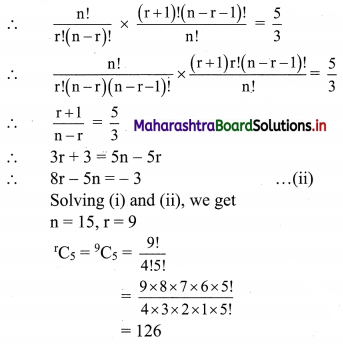Question 7.
Find the number of ways of drawing 9 balls from a bag that has 6 red balls, 5 green balls and 7 blue balls so that 3 balls of every colour are drawn.
Solution:
9 balls are to be selected from 6 red, 5 green, 7 blue balls such that the selection consists of 3 balls of each colour.
∴ 3 red balls can be selected from 6 red balls in 6C3 ways.
3 green balls can be selected from 5 green balls in 5C3 ways.
3 blue balls can be selected from 7 blue balls in 7C3 ways.
∴ Number of ways selection can be done if the selection consists of 3 balls of each colour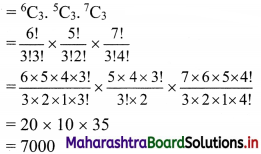Question 8.
Find the number of ways of selecting a team of 3 boys and 2 girls from 6 boys and 4 girls.
Solution:
There are 6 boys and 4 girls.
A team of 3 boys and 2 girls is to be selected.
∴ 3 boys can be selected from 6 boys in 6C3 ways.
2 girls can be selected from 4 girls in 4C2 ways.
∴ Number of ways the team can be selected = 6C3 × 4C2
= $$\frac{6 !}{3 ! 3 !} \times \frac{4 !}{2 ! 2 !}$$
= $$\frac{6 \times 5 \times 4 \times 3 !}{3 \times 2 \times 1 \times 3 !} \times \frac{4 \times 3 \times 2 !}{2 \times 2 !}$$
= 20 × 6
= 120
∴ The team of 3 boys and 2 girls can be selected in 120 ways.Question 9.
After a meeting, every participant shakes hands with every other participants. If the number of handshakes is 66, find the number of participants in the meeting.
Solution:
Let there be n participants present in the meeting.
A handshake occurs between 2 persons.
∴ Number of handshakes = nC2
Given 66 handshakes were exchanged.
∴ 66 = nC2
∴ 66 = $$\frac{n !}{2 !(n-2) !}$$
∴ 66 × 2 = $$\frac{\mathrm{n}(\mathrm{n}-1)(\mathrm{n}-2) !}{(\mathrm{n}-2) !}$$
∴ 132 = n(n – 1)
∴ n(n – 1) = 12 × 11
Comparing on both sides, we get n = 12
∴ 12 participants were present at the meeting.

Question 10.
If 20 points are marked on a circle, how many chords can be drawn?
Solution:
To draw a chord we need to join two points on the circle.
There are 20 points on a circle.
∴ Total number of chords possible from these points = 20C2
= $$\frac{20 !}{2 ! 18 !}$$
= $$\frac{20 \times 19 \times 18 !}{2 \times 1 \times 18 !}$$
= 190

Question 11.
Find the number of diagonals of an n-sided polygon. In particular, find the number of diagonals when
(i) n = 10
(ii) n = 15
(iii) n = 12
Solution:
In n-sided polygon, there are ‘n’ points and ‘n’ sides. .
∴ Through ‘n’ points we can draw nC2 lines including sides.
∴ Number of diagonals in n sided polygon = nC2 – n (∴ n = number of sides)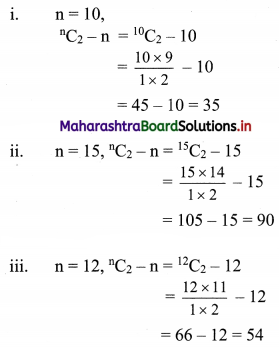Question 12.
There are 20 straight lines in a plane so that no two lines are parallel and no three lines are concurrent. Determine the number of points of intersection.
Solution:
There are 20 lines such that no two of them are parallel and no three of them are concurrent.
Since no two lines are parallel
∴ they intersect at a point
∴ Number of points of intersection if no two lines are parallel and no three lines are concurrent = 20C2
= $$\frac{20 !}{2 ! 18 !}$$
= $$\frac{20 \times 19 \times 18 !}{2 \times 1 \times 18 !}$$
= 190Question 13.
Ten points are plotted on a plane. Find the number of straight lines obtained by joining these points if
(i) no three points are collinear
(ii) four points are collinear
Solution:
There are 10 points on a plane.
(i) No three of them are collinear:
Since a line is obtained by joining 2 points,
number of lines passing through these points if no three points are collinear = 10C2
= $$\frac{10 !}{2 ! 8 !}$$
= $$\frac{10 \times 9 \times 8 !}{2 \times 1 \times 8 !}$$
= 5 × 9
= 45

(ii) When 4 of them arc collinear:
∴ Number of lines passing through these points if 4 points are collinear
= 10C24C2 + 1
= 45 – $$\frac{4 !}{2 ! 2 !}$$ + 1
= 45 – $$\frac{4 \times 3 \times 2 !}{2 \times 2 !}$$ + 1
= 45 – 6 + 1
= 40

Question 14.
Find the number of triangles formed by joining 12 points if
(i) no three points are collinear
(ii) four points are collinear
Solution:
There are 12 points on the plane
(i) When no three of them are collinear:
Since a triangle can be drawn by joining any three non-collinear points.
∴ Number of triangles that can be obtained from these points = 12C3
= $$\frac{12 !}{3 ! 9 !}$$
= $$\frac{12 \times 11 \times 10 \times 9 !}{3 \times 2 \times 1 \times 9 !}$$
= 220

(ii) When 4 of these points are collinear:
∴ Number of triangles that can be obtained from these points = 12C34C3
= 220 – $$\frac{4 !}{3 ! \times 1 !}$$
= 220 – $$\frac{4 \times 3 !}{3 !}$$
= 220 – 4
= 216Question 15.
A word has 8 consonants and 3 vowels. How many distinct words can be formed if 4 consonants and 2 vowels are chosen?
Solution:
Out of 8 consonants, 4 can be selected in 8C4
= $$\frac{8 !}{4 ! 4 !}$$
= $$\frac{8 \times 7 \times 6 \times 5 \times 4 !}{4 \times 3 \times 2 \times 1 \times 4 !}$$
= 70 ways
From 3 vowels, 2 can be selected in 3C2
= $$\frac{3 !}{2 ! 1 !}$$
= $$\frac{3 \times 2 !}{2 !}$$
= 3 ways
Now, to form a word, these 6 letters (i.e., 4 consonants and 2 vowels) can be arranged in 6P6 i.e., 6! ways.
∴ Total number of words that can be formed = 70 × 3 × 6!
= 70 × 3 × 720
= 151200
∴ 151200 words of 4 consonants and 2 vowels can be formed.